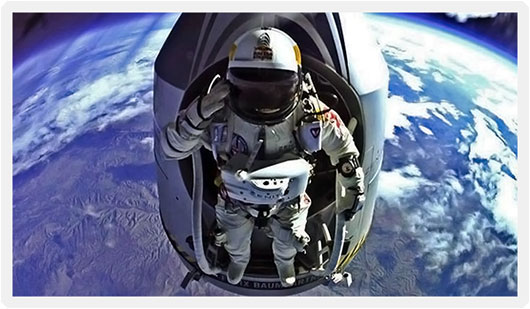# Fallen from Outer Space…# Record-Breaking Space Dive

14th October 2012.  During his ultimate high-altitude parachute jump, Felix Baumgartner spends approximately 4 minutes and 22 seconds in freefall, at the maximum speed of 1,342 kilometres per hour.

He is the first man ever to break the sound barrier without mechanical assistance.

In freefall, Felix travelled an astonishing distance.

## The distance travelled by a falling object is$d=\frac{1}{2}gt^2$

So,$d_{free fall}=\frac {9.81ms^{-2}\times(4\times60+22)s^{2}}{2} = 33,670 metres$

After setting the record for the highest manned balloon flight, and jumping from a record 39,045 metres into the stratosphere, he landed safely on both legs in the eastern desert of New Mexico.  Mission accomplished!  😀

Watch him get ready and jump from his capsule below.  Never ceases to amaze me…

# Gravitational Potential Energy

## Newton’s Law of Universal Gravitation is$\boldsymbol{F_{grav}}=\frac {-Gm_{1}m_{2}}{r^{2}}$

## The Gravitational Potential Energy of two masses is$E_{grav}=-\frac {Gm_{1}m_{2}}{r}$

For example, the Earth-Moon system has a gravitational potential energy

####$E_{grav_{Earth-Moon}} = - \frac {GM_{Earth}M_{Moon}}{r}$$E_{grav_{Earth-Moon}} = - \frac {GM_{Earth}M_{Moon}}{r}$.$E_{grav_{Earth-Moon}}=-\frac {6.7\times10^{-11}Nm^{2}kg^{-2}\times6.0\times10^{24}kg\times7.4\times10^{22}kg}{3.8\times10^{8}m}=-7.8\times10^{28}J$

Unfortunately, Felix does not appear to have released his precise vital statistics on the web.  A reasonable estimate has to be taken.  Say he’s about 160 pounds.  Overall, that gives $m_{Felix}=120 kg$.$E_{grav_{Earth-Felix}}=-\frac {6.7\times10^{-11}Nm^{2}kg^{-2}\times6.0\times10^{24}kg\times1.2\times10^{2}kg}{3.9\times10^{4}}=1.2 \times 10^{12} Joules$